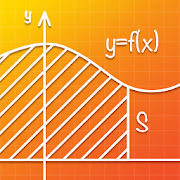# Graphing Calculator And Equation Solver CalculatorEveryone
38
Are you having trouble math graphing calculator? Are you afraid of solve math equations? Do not worried! Our graph mathematics app will help you!

As soon as you enter the calculation, the math equation solver application will graph immediately. In addition, the equation solver calculator application also supports some extremely useful tools. Please discover it yourself.

Features of free graph calculator app
👉 Math solve equation
👉 Solving the disequations
👉 Math graph solver
👉 Support many useful tools to solve math problem
👉 Save graph math solver
👉 Share math solve equation solution with friends

Highlights of calculator to solve equation app
🍀 Correct math equation solver
🍀 Quickcalculator math solver
🍀 Instant graphing calculator math pro
🍀 Easy to use graphing calculator app
🍀 Small maths solver app capacity
🍀 Free maths solution app

The mathematics solver application is completely free but offers a great experience. Let's start solving math and graphing now!

Don't forget to introduce the mathematics solve problem app to your friends so they can solve math and graph easily!

Please rate 5* to support the developer!
Collapse

## Reviews

Review Policy
3.2
38 total
5
4
3
2
1

Updated
July 12, 2019
Size
17M
Installs
1,000+
Current Version
1.0.2
Requires Android
5.0 and up
Content Rating
Everyone
Interactive Elements
Users Interact
Permissions
Offered By